Thermometers are useful apparatus being used since long time for temperature measurement. In this project we have made an Arduino based digital thermometer to display the current ambient temperature on a 16x2 LCD unit in real time . It can be deployed in houses, offices, industries etc. to measure the temperature. We can divide this Arduino based thermometer into three sections - The first section senses the temperature by using temperature sensor LM35, second section converts the temperature value into a suitable numbers in Celsius scale which is done by Arduino, and last part of system displays temperature on 16x2 LCD. The same is demonstrated in below block diagram.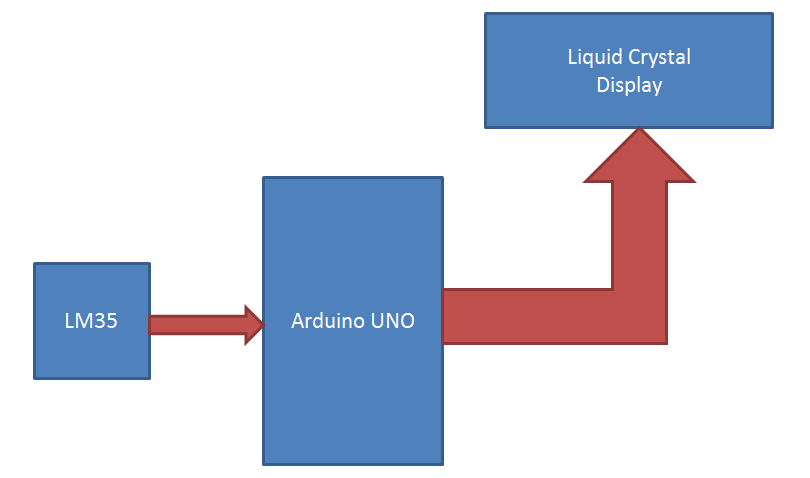In this digital temperature sensor with Arduino, Arduino Uno is used to control the whole process. An LM35 temperature sensor is used for sensing environment temperature which gives 1 degree temperature on every 10mV change at its output pin. You can easily check it with voltmeter by connecting Vcc at pin 1 and Ground at pin 3 and output voltage at pin 2 of LM35 sensor. For an example if the output voltage of LM35 sensor is 250m volt, that means the temperature is around 25 degree Celsius.

Arduino reads output voltage of temperature sensor by using Analog pin A0 and performs the calculation to convert this Analog value to a digital value of current temperature. After calculations arduino sends these calculations or temperature to 16x2 LCD unit by using appropriate commands of LCD. We have also built other digital thermometer projects using DHT11, DS18B20 and other temperature sensors.

### Circuit Components

Arduino

In this project we have used a Arduino to control whole the process of system. Arduino is a controller which runs on ATmega AVR controller. Arduino is an open source hardware platform and very useful for project development purpose. There are many types of Arduino boards like Arduino UNO, arduino mega, arduino pro mini, Lilypad etc. available in the market or you can also build Arduino by yourself.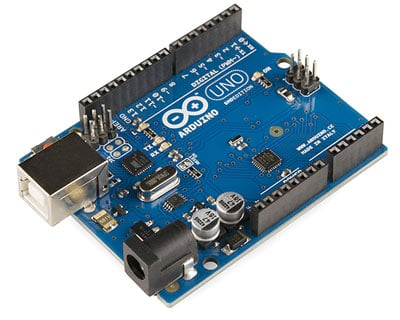LM35 Temperature Sensor

LM35 is a 3 pin temperature sensor which gives 1 degree Celsius on every 10mVolt change. This sensor can sense up to 150 degree Celsius temperature. 1 number pin of lm35 sensor is Vcc, second is output and third one is Ground. LM35 is the most simplest temperature sensor and can be interfaced easily with any microcontroller. You can check various Temperature Measurement using LM35 based projects here.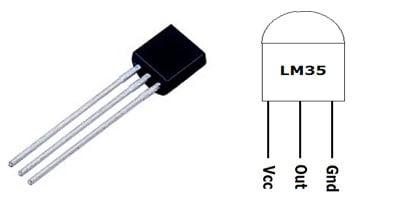Pin No Function Name 1 Supply voltage; 5V (+35V to -2V) Vcc 2 Output voltage (+6V to -1V) Output 3 Ground (0V) Ground

LM35 can be easily interfaced with Raspberry Pi, NodeMCU, PIC microcontroller, etc to measure the temperature and can also be used standalone with Op-amp to indicate temperature levels.

LCD

16x2 LCD unit is widely using in embedded system projects because it is cheap, easily availablet, small in size and easy to interface. 16x2 have two rows and 16 columns, which means it consist 16 blocks of 5x8 dots. 16 pin for connections in which 8 data bits D0-D7 and 3 control bits namely RS, RW and EN. Rest of pins are used for supply, brightness control and for backlight.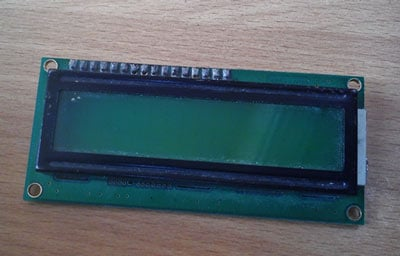Power Supply

Arduino Board already have an inbuilt power supply section. Here we only need to connect a 9 volt or 12 volt adaptors with the board.

### Circuit Diagram and Explanation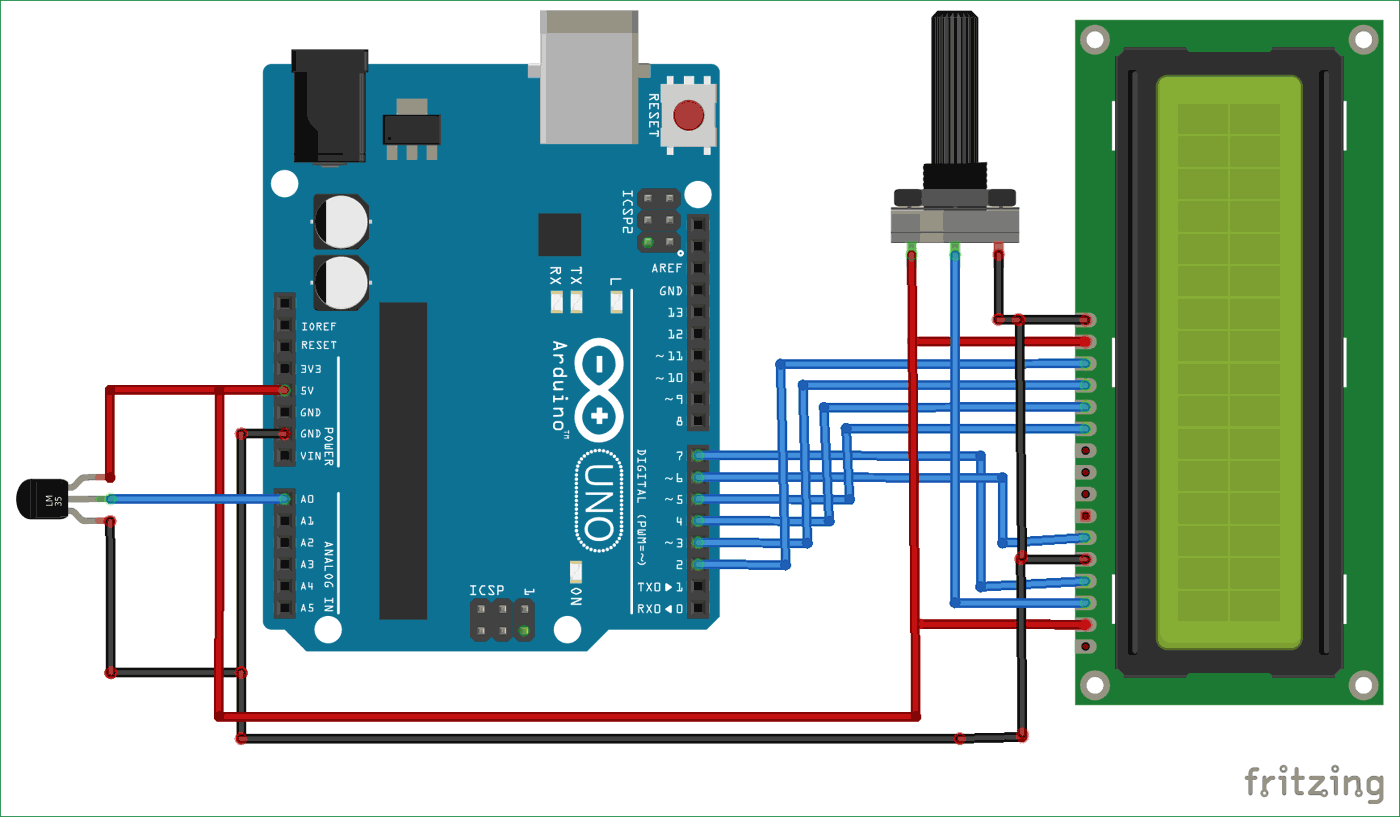Circuit digram for Digital Thermometer using Arduino and LM35 Temperature Sensor​, is shown in the above figure. Make the connections carefully as shown in the schematic. Here 16x2 LCD unit is directly connected to arduino in 4-bit mode. Data pins of LCD namely RS, EN, D4, D5, D6, D7 are connected to arduino digital pin number 7, 6, 5, 4, 3, 2. A temperature sensor LM35 is connected to Analog pin A0 of arduino, which generates 1 degree Celsius temperature on every 10mV output change at its output pin.

If you are new to Arduino then learn to interface 16x2 LCD with Arduino in our previous tutorial.

### Arduino LM35 Code & Explanation

The code for Temperature Measurement using LM35 is simple and given at the end of this tutorial. First we include library for LCD unit and then we defines data and control pins for LCD and temperature sensor.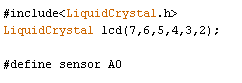After getting analog value at analog pin we reads that value using Analog read function and stores that value in a variable. And then convert the value into temperature by applying the below given formula.

```float analog_value=analogRead(analog_pin);
float Temperature=analog_value*factor*100
where
factor=5/1023
analog_value= output of temperature sensor```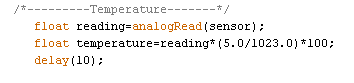Here degree symbol is created using custom character method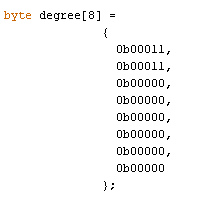So this is how we can build a simple Digital Thermometer to measure temperature with Arduino. Complete code with a demo video is given below.

Code

/*-----------Arduino LM35 Code-------------*/

/*-----------Digital Thermometer Using Arduino-------------*/

#include<LiquidCrystal.h>
LiquidCrystal lcd(7,6,5,4,3,2);

#define sensor A0

byte degree =
{
0b00011,
0b00011,
0b00000,
0b00000,
0b00000,
0b00000,
0b00000,
0b00000
};

void setup()
{
lcd.begin(16,2);
lcd.createChar(1, degree);
lcd.setCursor(0,0);
lcd.print("    Digital    ");
lcd.setCursor(0,1);
lcd.print("  Thermometer   ");
delay(4000);
lcd.clear();
lcd.print(" Circuit Digest  ");
delay(4000);
lcd.clear();
}

void loop()
{
/*---------Temperature-------*/
delay(10);

/*------Display Result------*/
lcd.clear();
lcd.setCursor(2,0);
lcd.print("Temperature");
lcd.setCursor(4,1);
lcd.print(temperature);
lcd.write(1);
lcd.print("C");
delay(1000);
}

Video

Subscribe below to receive most popular news, articles and DIY projects from Circuit Digest

### Related Content

•QAMAR SHAIKH
Dec 18, 2015

TRIED WORKING VERY GOOD

•harish
May 17, 2016

how to create your own programme code sir
i want to make new project
and how to understand that code line
pls tell that

•harish
May 18, 2016

it is a correct temperature or not

•Jul 17, 2016

It is very good and must project.Thank you sir.

•Hariprasath
Sep 25, 2016

Hello there ,
I am using an lm35 dz and arduino r3. My readings are varied from 0 to 500 degree celcius. What should i do for this.

Oct 07, 2016

Have you followed the code properly? Apply the given formula to convert the Analog temp reading into Degree C.

•shafqat Riaz
Jul 15, 2018

i have same problem with it

• thummar
Jan 20, 2019

Dear sir,

please tell me the soluion if you get a correct ans.

my problem is that if i not connect the sensor to the arduno uno however arduno generat temoreture in -18 to 500 c range.

warm regard

(=918758236036)

• Aswinth Raj
Jan 21, 2019

Hi,

You are not supposed to read anything from a pin if it is not connected to anything. If the pin is left free, it is said to be in floating condition and the value read from it can be anything from 0 toso yes there is no suprise that you are getting random calues as output.

If you want to read zero, use a pull down resistor. That is connect a 10 resistor from the analog pin to the ground pin and you will be able to read zero

•Jenan
Jan 29, 2017

good project

•Gainji GM
Mar 20, 2017

Sir here is a code for Arduino based digital thermometer how can i add buzzer to alert if temperature increase
(if temperature >50) then buzzer beeps

#include<LiquidCrystal.h>
LiquidCrystal lcd(2,4,7,8,9,10);

const int Sensor = A0;
byte degree_symbol =
{
0b00111,
0b00101,
0b00111,
0b00000,
0b00000,
0b00000,
0b00000,
0b00000
};
void setup()
{
pinMode(Sensor, INPUT);
lcd.begin(16,2);
lcd.createChar(1, degree_symbol);
lcd.setCursor(0,0);
lcd.print(" Digital ");
lcd.setCursor(0,1);
lcd.print(" Thermometer ");
delay(4000);
lcd.clear();
}
void loop()
{

delay(10);

lcd.clear();
lcd.setCursor(0,0);
lcd.print("Temperature in C");
lcd.setCursor(4,1);
lcd.print(temperature);
lcd.write(1);
lcd.print("C");
delay(1000);
}

•Anna
Mar 26, 2017

gd eve sir ! I am beginner in using arduino.Could I ask what codes i should add if I put a beep sound /alarm buzzer to alert if temperature increase.. thank you so much sir.

•Eranna
Mar 31, 2017

I tried this project n got output ...plz ping code for buzzer also

•karamjeet
Apr 03, 2017

I am not getting the output on lcd

•Speedfox
Aug 11, 2017

I want to make one thermometer like that but some different things, like using an arduino mini, extend the range and seal the temp sensor (to put it on boiling water) and add a computer speaker to know when you reach a determinate temp. do you think that can be possible?

•Mandaza
Oct 09, 2017

if the code is incorrect ,errors been detected what should l do

•sukhbir
Apr 11, 2018

What is A E S shown below the LCD

• Aswinth Raj
Apr 15, 2018

It is a potentiometer, it can be used to adjust the contract of the LCD. The pins AES are the three pins on a potentiometer respectively

•la4yt
Jun 15, 2018

How to get view with just one digit after,

•EMILY
Aug 27, 2018

someone got the buzzer code?